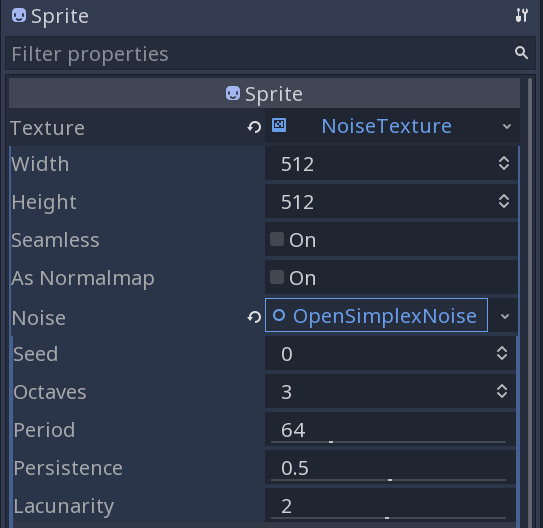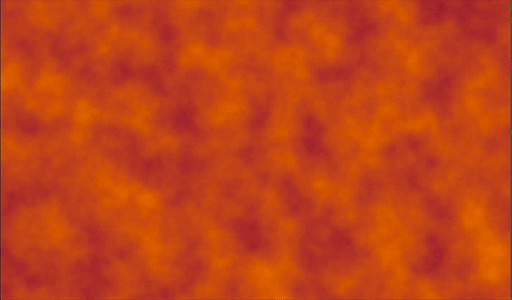# Noise

## Problem

You want to use Godot’s `OpenSimplexNoise` to generate noise, noise textures, and other effects.

## Solution

Noise, or more specifically gradient noise is a method for generating more natural-looking “random” patterns. To do this, Godot provides the OpenSimplexNoise class.

`OpenSimplexNoise` works by generating a 3D “cloud” of points. Each point has a value ranging from `-1` to `1`. Below are two examples of noise generated by `OpenSimplexNoise`. In the images, each pixel’s white value is mapped to the noise value at that point.The parameters used for configuring OpenSimplexNoise:

• `seed` - The seed used to generate the random values. Default value: `0`.
• `period` - Lower values result in higher frequency noise (i.e. more changes in value across the same distance). Default value: `64`.
• `octaves` - The number of noise layers that contribute to the result. Each successive layer affects the result less (based on the `persistence`). Default value: `3`.
• `lacunarity` - The difference in period between octaves. Default value: `2`.
• `persistence` - The amount that each octave contributes to the noise. Default value: `0.5`.

Here’s the easiest way to experiment with the effects of the different parameters. Add a `Sprite` and in its Texture property, choose “New NoiseTexture”. In that `NoiseTexture` for Noise choose “New OpenSimplexNoise”.You’ll see a black-and-white texture that’s generated based on the noise values. Adjust the values and observe the effect on the image.

### Using noise values

Once you have your noise configured, there are a few ways you can access the values.

• `get_noise_2d(x, y)`
• `get_noise_3d(x, y, z)`
• `get_noise_4d(x, y, z, w)`

In each case, the result is a value in the range `[-1, 1]`.

For example, we can use `get_noise_3d()` to generate a 2d image while varying the third dimension over time to get an animated effect:In this image the color is based on the noise value. Since we don’t want negative values for color, we use

`var value = get_noise_3d(x, y, z) * 0.5 + 0.5`

to modify the results to the range `[0, 1]`. Then, this value is applied to color using the following formula:

`Color(1.0-value/2.0, 0.5-value/2.0, value/4.0)`

### Noise applications

Noise can be used for a wide range of applications:

• Procedural terrain generation
• Visual effects (water, fog, fire, etc)